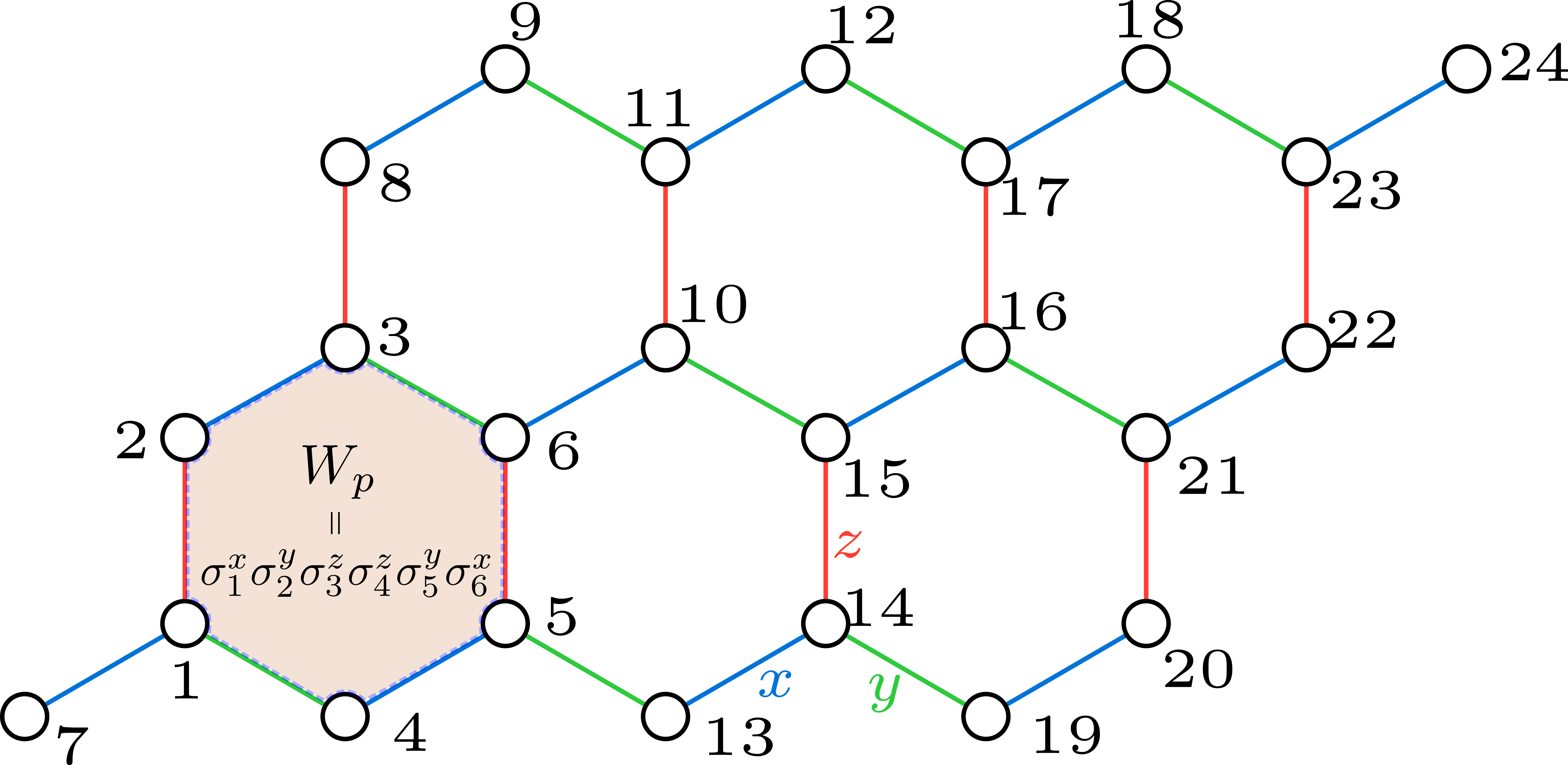# Measure multi-point correlation in 2D lattice

+1 vote

Hi everyone

Recently I've been trying to calculate some observable in Kitaev honeycomb model (Kitaev 2006) using ITensor DMRG. One important result to check out is the flux operator Wp whose ground state expectation should be Wp = +1 as calculated analytically by Kitaev himself.

However, I found that sometimes I cannot retrieve this number in dmrg. For example, in a 24-site system shown below:following the tutorial and other advices, the multi-point correlation for [s2 s3 s4 s7 s8 s9] is calculated using the following code:

    ITensor Sz_2 = op(sites, "Sx", 2);
ITensor Sz_3 = op(sites, "Sy", 3);
ITensor Sz_4 = op(sites, "Sz", 4);
ITensor Sz_7 = op(sites, "Sz", 7);
ITensor Sz_8 = op(sites, "Sy", 8);
ITensor Sz_9 = op(sites, "Sx", 9);

psi.position(2);

ITensor C = psi(2);
C *= Sz_2;  // with primed physical index
C *= dag(prime(prime(psi(2), "Site"), ir));

C *= psi(3);
C *= Sz_3;

C *= psi(4);
C *= Sz_4;

C *= psi(5);

C *= psi(6);

C *= psi(7);
C *= Sz_7;

C *= psi(8);
C *= Sz_8;

C *= psi(9);
C *= Sz_9;
C *= dag(prime(prime(psi(9), "Site"), il));

auto results = eltC(C * 64);  // spin-1/2 to pauli matrix by 2^6


where I have contracted site 5,6 directly since there's no operator acting on them. But this gives a wrong number Wp = -0.18 instead of +1; even though all 2-site operator are correct.

On the other hand, if I reorder the site indices so that the first 6 continuous sites in MPS correspond to the Wp operator, as shown below:where Wp = [s1 s2 s3 s4 s5 s6], then I got the correct expectation Wp = +1 by the same token.

It is curious to me that the order of sites makes such a huge difference, though both ordering gave the same ground state energy. I would like to understand the crux of this problem (maybe I missed some trivial stuff) before I implement more nested correlators in dmrg.

Could someone give any suggestions? Thank you.